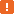# wValue Property (Specific to the Ext JS Time Field Control)This property is not supported in web tests (including cross-platform web tests) that use XPath expressions and CSS selectors to locate web elements. This property can be only used in tests that locate web objects by using internal identification properties provided by TestComplete.

### Description

Use this property to get the value that is currently displayed in the time field control or to set a new one.

### Declaration

TestObj.wValue

 TestObj A variable, parameter or expression that specifies a reference to one of the objects listed in the Applies To section

### Applies To

The property is applied to the following object:

### View Mode

This property is available in the Object Browser panel and in other panels and dialogs in both Basic and Advanced view modes.

### Property Value

The time currently selected in the control. The following types are possible:

Type Description
Date/Time A Date/Time value that contains a time portion.
String A string in one of time formats.
JavaScript (or JScript) Date object A JavaScript (or JScript) Date object that contains a time portion.

### Remarks

If the control’s time interval is limited by the native `minValue` and `maxValue` properties, `wValue` must be within this range.

The format of `wValue` depends on the native `format` property. The default format is "h":"mm AMPM", for example, "3:00 AM".Due to certain specifics of the Ext JS Time Field control, you cannot pass values containing the seconds part to the `wValue` property. For example, `wValue = "0:21:36"` sets "12:21 AM", fails and posts an error message.

### Example

The following example demonstrates how to obtain and set the time displayed in a control:

JavaScript, JScript

function Main()
{
var page = Sys.Browser().Page("http//www.example.com");
var TimeField = page.Panel("form").Table("timefield");

// Obtain the current value of the Time Field control
Log.Message(TimeField.wValue);

// Set the value of the Time Field using String data type
TimeField.wValue = "10.00 AM";

//Set the value of the Time Field using Date/Time data type
TimeField.wValue = 0.125;

// Set the value of the Time Field using JScript Date object
TimeField.wValue = new Date(null, null, null, 05, 25);
}

Python

``````def Main():
page = Sys.Browser().Page("http//www.example.com")
TimeField = page.Panel("form").Table("timefield")

# Obtain the current value of the Time Field control
Log.Message(TimeField.wValue)

# Set the value of the Time Field using String data type
TimeField.wValue = "10.00 AM"

# Set the value of the Time Field using Date/Time data type
TimeField.wValue = 0.125``````

VBScript

Sub Main
Set Page = Sys.Browser.Page("http://www.example.com")
Set TimeField = Page.Panel("form").Table("timefield")

' Obtain the current value of the Time Field control
Log.Message TimeField.wValue

' Set the value of the Time Field using String data type
TimeField.wValue = "11:00 AM"

' Set the value of the Time Field using Date/Time data type
TimeField.wValue = 0.125
End Sub

DelphiScript

procedure Main;
var page, TimeField;
begin
page := Sys.Browser.Page('http://www.example.com');
TimeField := page.Panel('form').Table('timefield');

// Obtain the current value of the Time Field control
Log.Message(TimeField.wValue);

// Set the value of the Time Field using String data type
TimeField.wValue := '10.00 AM';

// Set the value of the Time Field using Date/Time data type
TimeField.wValue := 0.125;
end;

C++Script, C#Script

function Main()
{
var page, TimeField
page = Sys["Browser"]()["Page"]("http://www.example.com");
TimeField = page["Panel"]("form")["Table"]("timefield");

// Obtain the current value of the Time Field control
Log["Message"](TimeField["wValue"]);

// Set the value of the Time Field using String data type
TimeField["wValue"] = "10.00 AM";

// Set the value of the Time Field using Date/Time data type
TimeField["wValue"] = 0.125;

// Set the value of the Time Field using JScript Date object
TimeField["wValue"] = new Date(null, null, null, 05, 25);
}